# Escape Velocity, it’s Formula and Derivation

When an object is thrown vertically upwards, it reaches a certain height and comes back to the earth. But when it is given greater initial velocity, it reaches greater height before coming back.

An object can be thrown up with a certain minimum initial velocity so that, the object goes beyond the earth’s gravitational field and escape from earth, this velocity is known as escape velocity of the earth. So, escape velocity is defined as the minimum initial velocity that will take a body away above the surface of a planet when it’s projected vertically upwards.

On throwing the object upwards, work has to be done against the gravity. If a certain minimum velocity is given to an object, such that the work done against gravity from the planet’s surface to infinity(outside gravitational field of planet) is equal to the kinetic energy of the object, then it will not return back to the planet.

## Derivation of Escape Velocity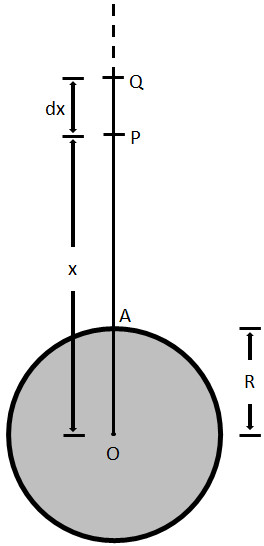Suppose that the planet be a perfect sphere of radius R having mass M. Let a body of mass m is to be projected from point A on the earth’s surface as shown in the figure. Join OA and produce it further. Let us take two points P and Q which are at distances x and (x + dx) from the center of the earth.

To calculate the escape velocity of the earth, let the minimum velocity to escape from the earth’s surface be ve. Then, kinetic energy of the object of mass m is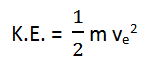When the projected object is at point P which is at a distance x from the center of the earth, the force of gravity between the object and earth is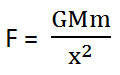Work done in taking the body against gravitational attraction from P to Q is given by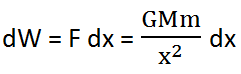The total amount of work done in taking the body against gravitational attraction from surface of the earth to infinity can be calculated by integrating the above equation within the limits x = R to x =. Hence, total work done is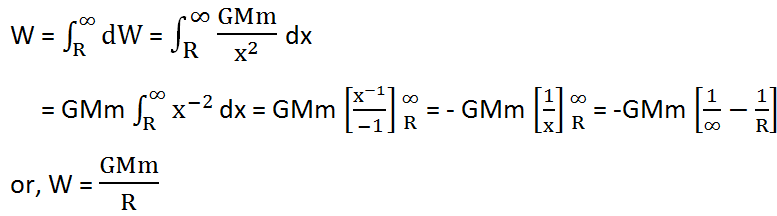For the object to escape from the earth’s surface, kinetic energy given must be equal to the work done against gravity going from the earth’s surface to infinity, hence

### K.E. of Object should be Equal to Magnitude of P.E.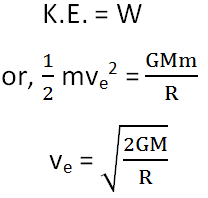Since,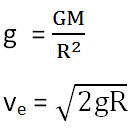The relation shows that the escape velocity of an object does not depend on the mass of the projected object but only on the mass and radius of the planet from which it is projected.

## Escape Velocity of Earth

For the earth, g = 9.8 m/s2 and R = 6.4 X 106 m, then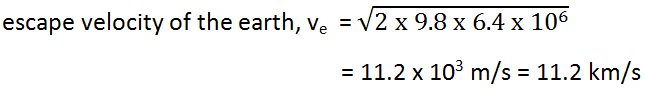That means, a spacecraft leaving earth surface should have 11.2 km/sec or 7 miles/sec initial velocity to escape from earth’s gravitational field.